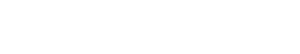# Skewness

Skewness means asymmetry in the distribution of a data set. If a data set is symmetrical, then the mean, median, and mode will be the same. However, if there is skewness in the data, then the mean, median, and mode will not be equal. Skewness can be positive or negative. Positive skewness means that the data are skewed to the right of the mean, and negative skewness means that the data are skewed to the left of the mean.

There are many ways to calculate skewness, but one of the most common is to use the difference between the mean and median divided by the standard deviation. This method is sometimes referred to as Pearson’s first coefficient of skewness.

Skewness is often used to identify outliers in a data set. Outliers are data points that are far from the rest of the data, and they can skew the results of an analysis. Skewness can also be used to characterize the shape of a distribution.

Skewness is just one measure of asymmetry. There are other measures, such as kurtosis and skew coefficient, that can be used to characterize the shape of a data set.

## Kurtosis vs Skew coefficient

Kurtosis is another measure of asymmetry. Kurtosis is a measure of the peakedness of a data set. The higher the kurtosis, the more peaked the data are. Skew coefficient is a measure of skewness that is less affected by outliers.

When comparing skewness to other measures of asymmetry, it is important to keep in mind that each measure has its own advantages and disadvantages. Skewness is a simple measure that is easy to calculate. However, it is affected by outliers, and it does not describe the entire distribution. Kurtosis is a more sophisticated measure that describes the entire distribution, but it is more difficult to calculate.

## Skewness is Outside of Text Analytics

There are many industries that use skewness as a measure of asymmetry. For example, in finance, skewness is used to describe the distribution of returns. In insurance, skewness is used to describe the distribution of claims. In manufacturing, skewness is used to describe the distribution of product defects.Unlock the power of actionable insights with AI-based natural language processing.